# Vary Mutation and Crossover

### Setting the Amount of Mutation

The genetic algorithm applies mutations using the `MutationFcn` option. The default mutation option, `@mutationgaussian`, adds a random number, or mutation, chosen from a Gaussian distribution, to each entry of the parent vector. Typically, the amount of mutation, which is proportional to the standard deviation of the distribution, decreases at each new generation. You can control the average amount of mutation that the algorithm applies to a parent in each generation through the `Scale` and `Shrink` inputs that you include in a cell array:

```options = optimoptions('ga',... 'MutationFcn',{@mutationgaussian Scale Shrink}); ```

`Scale` and `Shrink` are scalars with default values `1` each.

• `Scale` controls the standard deviation of the mutation at the first generation. This value is `Scale` multiplied by the range of the initial population, which you specify by the `InitialPopulationRange` option.

• `Shrink` controls the rate at which the average amount of mutation decreases. The standard deviation decreases linearly so that its final value equals 1 – `Shrink` times its initial value at the first generation. For example, if `Shrink` has the default value of 1, then the amount of mutation decreases to 0 at the final step.

You can see the effect of mutation by selecting the plot functions `@gaplotdistance` and `@gaplotrange`, and then running the genetic algorithm on a problem such as the one described in Minimize Rastrigin's Function. The following figure shows the plot after setting the random number generator.

```rng default % For reproducibility options = optimoptions('ga','PlotFcn',{@gaplotdistance,@gaplotrange},... 'MaxStallGenerations',200); % to get a long run [x,fval] = ga(@rastriginsfcn,2,[],[],[],[],[],[],[],options);```
```Optimization terminated: maximum number of generations exceeded. ```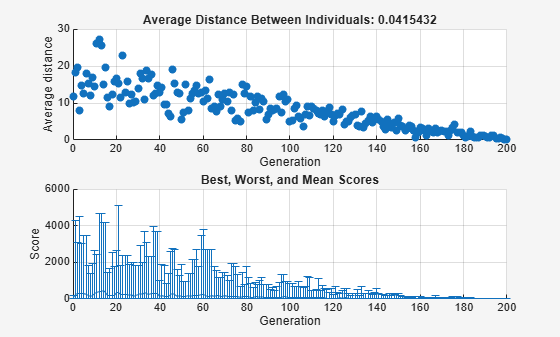The upper plot displays the average distance between points in each generation. As the amount of mutation decreases, so does the average distance between individuals, which is approximately 0 at the final generation. The lower plot displays a vertical line at each generation, showing the range from the smallest to the largest fitness value, as well as mean fitness value. As the amount of mutation decreases, so does the range. These plots show that reducing the amount of mutation decreases the diversity of subsequent generations.

For comparison, the following figure shows the same plots when you set `Shrink` to `0.5`.

```options = optimoptions('ga',options,... 'MutationFcn',{@mutationgaussian,1,.5}); [x,fval] = ga(@rastriginsfcn,2,[],[],[],[],[],[],[],options);```
```Optimization terminated: maximum number of generations exceeded. ```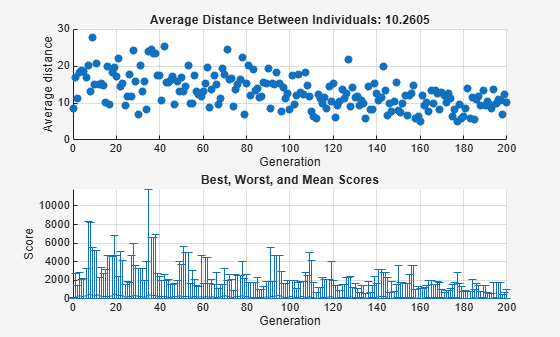This time, the average amount of mutation decreases by a factor of 1/2 by the final generation. As a result, the average distance between individuals decreases less than before.

### Setting the Crossover Fraction

The `CrossoverFraction` option specifies the fraction of each population, other than elite children, that are made up of crossover children. A crossover fraction of `1` means that all children other than elite individuals are crossover children, while a crossover fraction of `0` means that all children are mutation children. The following example show that neither of these extremes is an effective strategy for optimizing a function.

The example uses the fitness function whose value at a point is the sum of the absolute values of the coordinates at the points. That is,

`$f\left({x}_{1},{x}_{2},...,{x}_{n}\right)=|{x}_{1}|+|{x}_{2}|+\cdots +|{x}_{n}|.$`

You can define this function as an anonymous function by setting the fitness function to

`fun = @(x) sum(abs(x));`

Run the example with the default value of `0.8` as the `CrossoverFraction` option.

```nvar = 10; options = optimoptions('ga',... 'InitialPopulationRange',[-1;1],... 'PlotFcn',{@gaplotbestf,@gaplotdistance}); rng(14,'twister') % For reproducibility [x,fval] = ga(fun,nvar,[],[],[],[],[],[],[],options)```
```Optimization terminated: average change in the fitness value less than options.FunctionTolerance. ```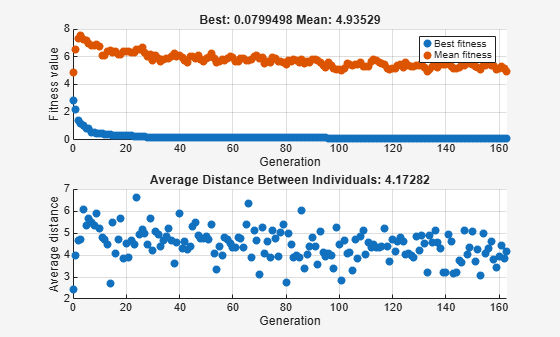```x = 1×10 -0.0020 -0.0134 -0.0067 -0.0028 -0.0241 -0.0118 0.0021 0.0113 -0.0021 -0.0036 ```
```fval = 0.0799 ```

#### Crossover Without Mutation

To see how the genetic algorithm performs when there is no mutation, set the `CrossoverFraction` option to `1.0` and rerun the solver.

```options.CrossoverFraction = 1; [x,fval] = ga(fun,nvar,[],[],[],[],[],[],[],options)```
```Optimization terminated: average change in the fitness value less than options.FunctionTolerance. ```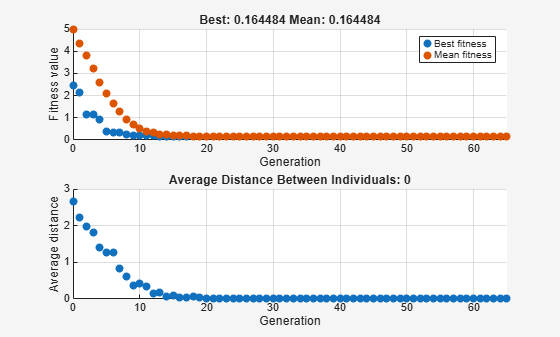```x = 1×10 -0.0275 -0.0043 0.0372 -0.0118 -0.0377 -0.0444 -0.0258 -0.0520 0.0174 0.0533 ```
```fval = 0.3114 ```

In this case, the algorithm selects genes from the individuals in the initial population and recombines them. The algorithm cannot create any new genes because there is no mutation. The algorithm generates the best individual that it can using these genes at generation number 8, where the best fitness plot becomes level. After this, it creates new copies of the best individual, which are then are selected for the next generation. By generation number 17, all individuals in the population are the same, namely, the best individual. When this occurs, the average distance between individuals is 0. Since the algorithm cannot improve the best fitness value after generation 8, it stalls after 50 more generations, because Stall generations is set to 50.

#### Mutation Without Crossover

To see how the genetic algorithm performs when there is no crossover, set the `CrossoverFraction` option to `0`.

```options.CrossoverFraction = 0; [x,fval] = ga(fun,nvar,[],[],[],[],[],[],[],options)```
```Optimization terminated: average change in the fitness value less than options.FunctionTolerance. ```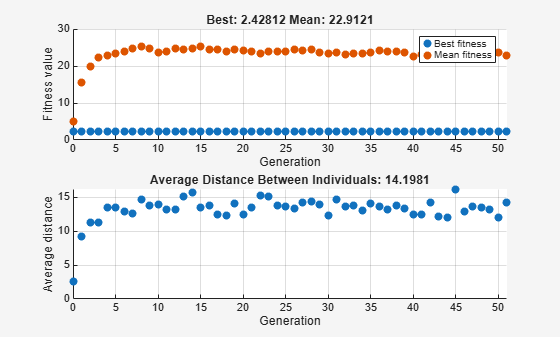```x = 1×10 0.4014 0.0538 0.7824 0.1930 0.0513 -0.4801 0.9988 -0.0059 0.0875 0.0302 ```
```fval = 3.0843 ```

In this case, the random changes that the algorithm applies never improve the fitness value of the best individual at the first generation. While it improves the individual genes of other individuals, as you can see in the upper plot by the decrease in the mean value of the fitness function, these improved genes are never combined with the genes of the best individual because there is no crossover. As a result, the best fitness plot is level and the algorithm stalls at generation number 50.

### Comparing Results for Varying Crossover Fractions

The example `deterministicstudy.m`, which is included when you run this example, compares the results of applying the genetic algorithm to Rastrigin's function with the `CrossoverFraction` option set to `0`, `0.2`, `0.4`, `0.6`, `0.8`, and `1`. The example runs for 10 generations. At each generation, the example plots the means and standard deviations of the best fitness values in all the preceding generations, for each value of the `CrossoverFraction` option.

Run the example.

`deterministicstudy`
```Optimization terminated: average change in the fitness value less than options.FunctionTolerance. Optimization terminated: average change in the fitness value less than options.FunctionTolerance. Optimization terminated: average change in the fitness value less than options.FunctionTolerance. Optimization terminated: average change in the fitness value less than options.FunctionTolerance. Optimization terminated: average change in the fitness value less than options.FunctionTolerance. Optimization terminated: average change in the fitness value less than options.FunctionTolerance. Optimization terminated: average change in the fitness value less than options.FunctionTolerance. Optimization terminated: average change in the fitness value less than options.FunctionTolerance. Optimization terminated: average change in the fitness value less than options.FunctionTolerance. Optimization terminated: average change in the fitness value less than options.FunctionTolerance. Optimization terminated: average change in the fitness value less than options.FunctionTolerance. Optimization terminated: average change in the fitness value less than options.FunctionTolerance. Optimization terminated: average change in the fitness value less than options.FunctionTolerance. Optimization terminated: average change in the fitness value less than options.FunctionTolerance. Optimization terminated: average change in the fitness value less than options.FunctionTolerance. Optimization terminated: average change in the fitness value less than options.FunctionTolerance. Optimization terminated: average change in the fitness value less than options.FunctionTolerance. Optimization terminated: average change in the fitness value less than options.FunctionTolerance. Optimization terminated: average change in the fitness value less than options.FunctionTolerance. Optimization terminated: average change in the fitness value less than options.FunctionTolerance. Optimization terminated: average change in the fitness value less than options.FunctionTolerance. Optimization terminated: average change in the fitness value less than options.FunctionTolerance. Optimization terminated: average change in the fitness value less than options.FunctionTolerance. Optimization terminated: average change in the fitness value less than options.FunctionTolerance. Optimization terminated: average change in the fitness value less than options.FunctionTolerance. Optimization terminated: average change in the fitness value less than options.FunctionTolerance. Optimization terminated: average change in the fitness value less than options.FunctionTolerance. Optimization terminated: average change in the fitness value less than options.FunctionTolerance. Optimization terminated: average change in the fitness value less than options.FunctionTolerance. Optimization terminated: average change in the fitness value less than options.FunctionTolerance. Optimization terminated: average change in the fitness value less than options.FunctionTolerance. Optimization terminated: average change in the fitness value less than options.FunctionTolerance. Optimization terminated: average change in the fitness value less than options.FunctionTolerance. Optimization terminated: average change in the fitness value less than options.FunctionTolerance. Optimization terminated: average change in the fitness value less than options.FunctionTolerance. Optimization terminated: average change in the fitness value less than options.FunctionTolerance. Optimization terminated: average change in the fitness value less than options.FunctionTolerance. Optimization terminated: average change in the fitness value less than options.FunctionTolerance. Optimization terminated: average change in the fitness value less than options.FunctionTolerance. Optimization terminated: average change in the fitness value less than options.FunctionTolerance. Optimization terminated: average change in the fitness value less than options.FunctionTolerance. Optimization terminated: average change in the fitness value less than options.FunctionTolerance. Optimization terminated: average change in the fitness value less than options.FunctionTolerance. Optimization terminated: average change in the fitness value less than options.FunctionTolerance. Optimization terminated: average change in the fitness value less than options.FunctionTolerance. Optimization terminated: average change in the fitness value less than options.FunctionTolerance. Optimization terminated: average change in the fitness value less than options.FunctionTolerance. Optimization terminated: average change in the fitness value less than options.FunctionTolerance. Optimization terminated: average change in the fitness value less than options.FunctionTolerance. Optimization terminated: average change in the fitness value less than options.FunctionTolerance. Optimization terminated: average change in the fitness value less than options.FunctionTolerance. Optimization terminated: average change in the fitness value less than options.FunctionTolerance. Optimization terminated: average change in the fitness value less than options.FunctionTolerance. Optimization terminated: average change in the fitness value less than options.FunctionTolerance. Optimization terminated: average change in the fitness value less than options.FunctionTolerance. Optimization terminated: average change in the fitness value less than options.FunctionTolerance. Optimization terminated: average change in the fitness value less than options.FunctionTolerance. Optimization terminated: average change in the fitness value less than options.FunctionTolerance. Optimization terminated: average change in the fitness value less than options.FunctionTolerance. Optimization terminated: average change in the fitness value less than options.FunctionTolerance. ```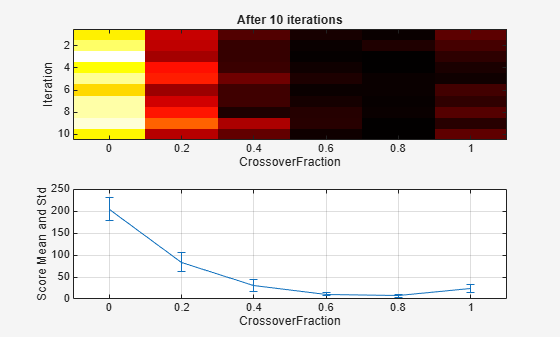The lower plot shows the means and standard deviations of the best fitness values over 10 generations, for each of the values of the crossover fraction. The upper plot shows a color-coded display of the best fitness values in each generation.

For this fitness function, setting Crossover fraction to `0.8` yields the best result. However, for another fitness function, a different setting for Crossover fraction might yield the best result.